## E.3 Signal processing

36.521-13GPPEvolved Universal Terrestrial Radio Access (E-UTRA)Part 1: Conformance testingRadio transmission and receptionRelease 17TSUser Equipment (UE) conformance specification

## E.3.1 Pre FFT minimization process

Before applying the pre-FFT minimization process, z(ν) and i(ν) are portioned into 20 pieces, comprising one slot each. Each slot is processed separately. Sample timing, Carrier frequency and carrier leakage in z(ν) are jointly varied in order to minimise the difference between z(ν) and i(ν). Best fit (minimum difference) is achieved when the RMS difference value between z(ν) and i(ν) is an absolute minimum.

The carrier frequency variation and the IQ variation are the measurement results: Carrier Frequency Error and Carrier leakage.

From the acquired samples 20 carrier frequencies and 20 carrier leakages can be derived.

NOTE 1: The minimisation process, to derive carrier leakage and RF error can be supported by Post FFT operations. However the minimisation process defined in the pre FFT domain comprises all acquired samples (i.e. it does not exclude the samples in between the FFT widths and it does not exclude the bandwidth outside the transmission bandwidth configuration

NOTE 2: The algorithm would allow deriving Carrier Frequency error and Sample Frequency error of the TX under test separately. However there are no requirements for Sample Frequency error. Hence the algorithm models the RF and the sample frequency commonly (not independently). It returns one error and does not distinguish between both.

After this process the samples z(ν) are called z0(ν).

## E.3.2 Timing of the FFT window

The FFT window length is 2048 samples per OFDM symbol. 7 FFTs (14336 samples) cover less than the acquired number of samples (15360 samples) The position in time for FFT must be determined.

In an ideal signal, the FFT may start at any instant within the cyclic prefix without causing an error. The TX filter, however, reduces the window. The EVM requirements shall be met within a window W<CP. There are three different instants for FFT:

Centre of the reduced window, called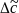,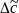–W/2 and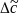+W/2.

The timing of the measured signal is determined in the pre FFT domain as follows, using z0(ν) and i2(ν) :

1. The measured signal is delay spread by the TX filter. Hence the distinct boarders between the OFDM symbols and between Data and CP are also spread and the timing is not obvious.

2. In the Reference Signal i2(ν) the timing is known.

3. Correlation between (1.) and (2.) will result in a correlation peak. The meaning of the correlation peak is approx. the “impulse response” of the TX filter. The meaning of “impulse response” assumes that the autocorrelation of the reference signal i2(ν) is a Dirac peak and that the correlation between the reference signal i2(ν) and the data in the measured signal is 0. The correlation peak, (the highest, or in case of more than one, the earliest) indicates the timing in the measured signal.

From the acquired samples 20 timings can be derived.

For all calculations, except EVM, the number of samples in z0(ν) is reduced to 7 blocks of samples, comprising 2048 samples (FFT width) and starting within each OFDM symbol including the demodulation reference signal.

For the EVM calculation the output signal under test is reduced to 14 blocks of samples, comprising 2048 samples (FFT width) and starting with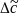–W/2 and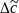+W/2 in each OFDM symbol including the demodulation reference signal.

The number of samples, used for FFT is reduced compared to z0(ν). This subset of samples is called z’(ν).

The timing of the centre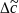with respect to the different CP length in a slot is as follows: (Frame structure 1, normal CP length)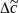is on Tf=72 within the CP of length 144 (in OFDM symbol 1 to 6)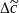is on Tf=88 (=160-72) within the CP of length 160 (in OFDM symbol 0)

## E.3.3 Post FFT equalisation

Perform 7 FFTs on z’(ν), one for each OFDM symbol in a slot using the timing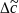, including the demodulation reference symbol. The result is an array of samples, 7 in the time axis t times 2048 in the frequency axis f. The samples represent the DFT coded data symbols (in OFDM-symbol 0,1,2,4,5and 6 in each slot) and demodulation reference symbols ( OFDM symbol 3 in each slot) in the allocated RBs and inband emissions in the non allocated RBs within the transmission BW.

Only the allocated resource blocks in the frequency domain are used for equalisation.

The nominal demodulation reference symbols and nominal DFT coded data symbols are used to equalize the measured data symbols. (Location for equalization see Figure E.2.5-1)

NOTE: The nomenclature inside this note is local and not valid outside.

The nominal DFT coded data symbols are created by a demodulation process. The location to gain the demodulated data symbols is “EVM” in Figure E.2.5-1. A demodulation process as follows is recommended:

1. Equalize the measured DFT coded data symbols using the reference symbols for equalisation. Result: Equalized DFT coded data symbols

2. iDFT transform the equalized DFT coded data symbols: Result: Equalized data symbols

3. Decide for the nearest constellation point: Result: Nominal data symbols

4. DFT transform the nominal data symbols: Result: Nominal DFT coded data symbols

At this stage we have an array of Measured DFT coded data-Symbols and reference-Symbols (MS(f,t))

versus an array of Nominal DFT coded data-Symbols and reference Symbols (NS(f,t))

(complex, the arrays comprise 6 DFT coded data symbols and 1 demodulation reference symbol in the time axis and the number of allocated subcarriers in the frequency axis.)

MS(f,t) and NS(f,t) are processed with a least square (LS) estimator, to derive one equalizer coefficient per time slot and per allocated subcarrier. EC(f)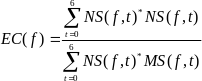With * denoting complex conjugation.

EC(f) are used to equalize the DFT-coded data symbols. The measured DFT-coded data and the references symbols are equalized by:

Z’(f,t) = MS(f,t) . EC(f)

With . denoting multiplication.

Z’(f,t), restricted to the data symbol (excluding t=3) is used to calculate EVM, as described in E.4.1.

EC(f) is used in E.4.4 to calculate EVM equalizer spectral flatness.

NOTE: although an exclusion period for EVM may be applicable in E.7, the post FFT minimisation process is done over 7 symbols (6 DFT-coded data symbols and 1 reference symbol).

The samples of the non allocated resource blocks within the transmission bandwidth configuration in the post FFT domain are called Y(f,t) (f covering the non allocated subcarriers within the transmission bandwidth configuration, t covering the OFDM symbols during 1 slot).